## 3 different methods for substring in javascript

Substring means a portion of a string. Substring is extracted based on numeric indexes. This article will explain 3 different ways in which a substring can be retrieved from a string in javascript.

Method 1: substr function
Javascript `substr` function takes two arguments with their meanings given below.
1. Start index: Index of character from which the substring will be extracted.
2. Count: Total number of characters or length of substring.
Syntax of `substr` function is

substr(index, length)

By default characters in a string start at index 0 from left to right. Below illustration explains the working of `substr` function in javascript.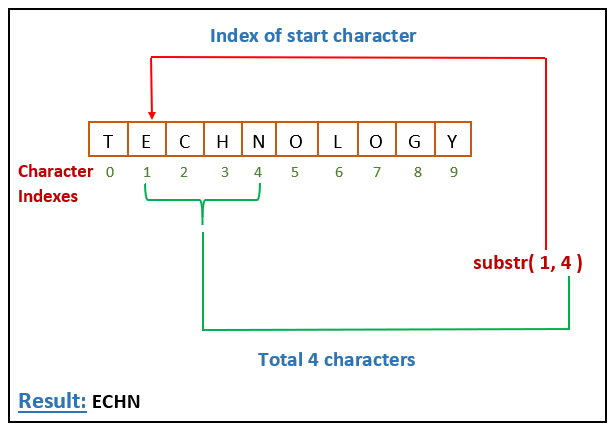Following points should be remembered regarding `substr` function.

• Both arguments or numeric values are optional.
• If no argument is present, then it considers the first one as 0 and returns the entire string as it is.
• If second argument(or length) is not present, then it returns characters till the end of string.
• If any argument is `NaN`, it is considered as 0.
• If second argument is greater than the length of the string, `substr` returns all the characters starting with the character at index at first argument value.
• Indexes of characters from right to left are negative. That is, index of first character at right is -1, second is -2 and so on.
Thus, if the first argument is negative, it will return the characters starting from right end or end of string.

Examples of `substr` function based on above pointers are given below.

```   let str = "Technology";
document.write("String:: " + str)
document.write('<br>------------------------<br>');
document.write("str.substr() => " + str.substr());
document.write('<br>');
document.write("str.substr(1, 5) => " + str.substr(1, 5));
document.write('<br>');
document.write("str.substr(NaN, 4) => " + str.substr(NaN, 4));
document.write('<br>');
document.write("str.substr(-3, 2) => " + str.substr(-3, 2));```

Above example will print below output on browser.

String:: Technology
————————
str.substr() => Technology
str.substr(1, 5) => echno
str.substr(NaN, 4) => Tech
str.substr(-3, 2) => og

Method 2: substring function
Javascript `substring` function accepts 2 numeric arguments. First is the start index and second is the end index and returns the string between the start and (end index – 1).
Working of `substring` can be understood with following illustration.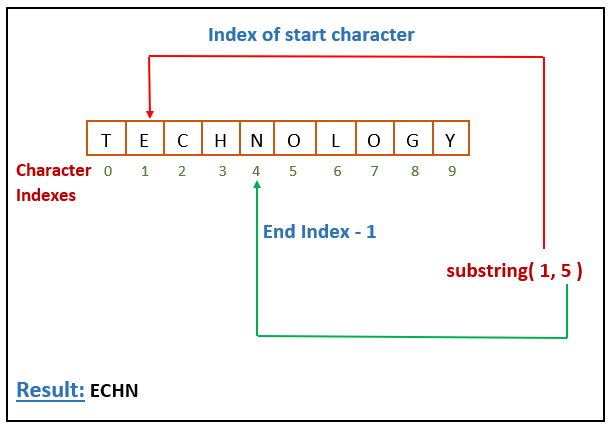Following points are worth considering for `substring` function.

• Both arguments are optional. If none is provided, `substring` returns the entire string.
• If second argument is omitted, `substring` returns all characters starting from first argument index till the end.
• If any argument is `NaN` or negative, it is considered as 0.
• If first argument(or start index) is greater than second argument(or end index), then both are swapped.
• If both are equal, `substring` will return an empty string.

Go through following examples for a better understanding of `substring` function.

```  let str = "Technology";
document.write("String:: " + str)
document.write('<br>------------------------<br>');
document.write("str.substring() => " + str.substring());
document.write('<br>');
document.write("str.substring(1, 5) => " + str.substring(1, 5));
document.write('<br>');
document.write("str.substring(NaN, 4) => " + str.substring(NaN, 4));
document.write('<br>');
document.write("str.substring(4, NaN) => " + str.substring(4, NaN));
document.write('<br>');
document.write("str.substring(-3, 2) => " + str.substring(-3, 2));
document.write('<br>');
document.write("str.substring(3, 0) => " + str.substring(3, 0));```

This code will print below output on browser window.

str.substring() => Technology
str.substring(1, 5) => echn
str.substring(NaN, 4) => Tech
str.substring(4, NaN) => Tech
str.substring(-3, 2) => Te
str.substring(3, 0) => Tec

Method 3: slice function
Javascript `slice` function is also used to extract a substring from a string. It accepts two numeric arguments where first argument is the start index and second argument is the end index and returns the string between those two indexes excluding the character at end index.
Following points should be remembered related to `slice` function.

• If no argument is provided, `slice` returns the entire string.
• If second argument(or end index) is not provided, `slice` will return substring from start index till the end of string.
• If second argument is greater than the length of the string, `slice` returns the string from start index till the end.

Go through below examples carefully to understand the usage of `slice` function.

```   let str = "Technology";
document.write("String:: " + str)
document.write('<br>------------------------<br>');
document.write("str.slice() => " + str.slice());
document.write('<br>');
document.write("str.slice(1, 5) => " + str.slice(1, 5));
document.write('<br>');
document.write("str.slice(1, 20) => " + str.slice(1, 20));
document.write('<br>');
document.write("str.slice(NaN, 4) => " + str.slice(NaN, 4));
document.write('<br>');
document.write("str.slice(4, NaN) => " + str.slice(4, NaN));```

Above code will output

String:: Technology
————————
str.slice() => Technology
str.slice(1, 5) => echn
str.slice(1, 20) => echnology
str.slice(NaN, 4) => Tech
str.slice(4, NaN) =>

Negative indexes in slices
As stated earlier, character index in a string start from 0. If the string is traversed from right to left, then the indexes are counted negative with the first character at the end having index -1, second as -2 and so on as shown below.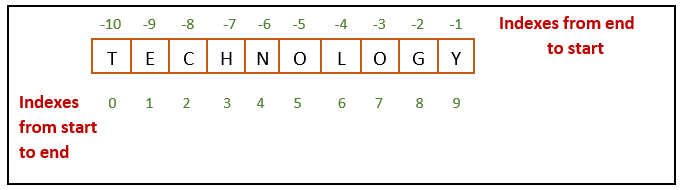`slice` supports negative indexes as per the following rules.

• If there is only one argument and it is negative, then it will start counting from the end till the given index. That is, `slice(-3)` will return the last three characters.
• If the second argument is negative, slice will return the substring between start index(from left to right) and end index counting from the end of the string.
Thus, “javascript”.slice(3, -5) will return “as”, the string between character at second index and third character from end excluding the third character as shown below.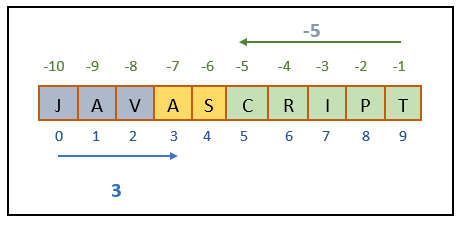Note that the character at start index is included while the character at end index is not included.
• If first argument is negative and second is positive, slice starts counting backwards from negative index(first argument) and forward from positive index(second argument) and returns the characters that fall between the two indexes as shown below.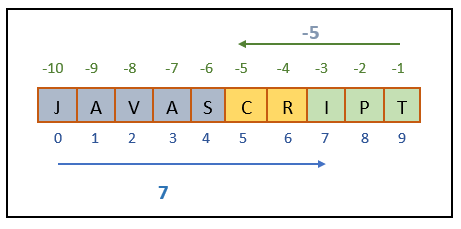“javascript”.slice(-5, 7) will return “cr”, characters between -5(counted backwards) and 7(counted from left to right). Note that character at second argument is excluded in this case also.

Below examples will be helpful in understanding how slice handles negative arguments.

```   let str = "Technology";
document.write("String:: " + str)
document.write('<br>------------------------<br>');
document.write("str.slice(-3) => " + str.slice(-3));
document.write('<br>');
document.write("str.slice(2, -3) => " + str.slice(2, -3));
document.write('<br>');
document.write("str.slice(-3, 9) => " + str.slice(-3, 9));
document.write('<br>');
document.write("str.slice(-3, 2) => " + str.slice(-3, 2));
document.write('<br>');
document.write("str.slice(-3, -1) => " + str.slice(-3, -1));```

Above code will output

String:: Technology
————————
str.slice(-3) => ogy
str.slice(2, -3) => chnol
str.slice(-3, 9) => og
str.slice(-3, 2) =>
str.slice(-3, -1) => og

substring Vs substr Vs slice
Though all the 3 functions are used to determine the substring but there is a difference between them. Below table summarizes the difference between these functions.

Areasubstrsubstringslice
Second argument meaningSecond argument is the count of characters that are included in substring.Second argument is the index till which characters will be included.Second argument is the index till which characters will be included.
Optional Second argumentSecond argument is optional.Second argument is optional.Second argument is optional.
WorkingReturns the characters from first argument till the total number of characters are equal to second argument.Returns the characters between first and second argument.
Character at first argument index is included and second argument index is excluded.
Returns the characters between first and second argument.
Character at first argument index is included and second argument index is excluded.
NaNAny argument as NaN is treated as 0.Any argument as NaN is treated as 0.Any argument as NaN is treated as 0.
Negative second argumentNegative second argument is treated as 0.Negative second argument is treated as 0.Negative second argument is counted from backwards.
First argument > Second argumentReturns the number of characters equal to second argument starting from first argument.Swaps the two arguments and returns the string between them.Returns empty string.
First argument = Second argumentReturns the number of characters equal to second argument starting from first argument.Returns empty string.Returns empty string.

Do not forget to hit the clap if the article was useful.

0
Liked the article ? Spread the word...